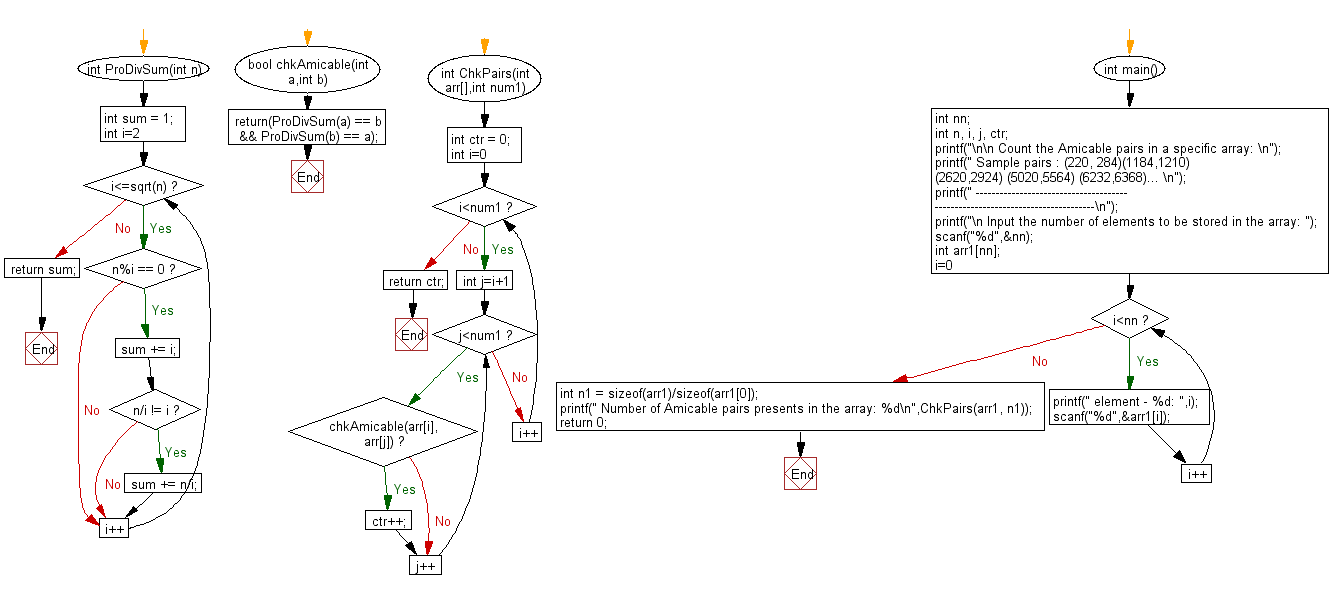﻿ C Program: Count the amicable pairs in an array - w3resource# C Exercises: Count the amicable pairs in an array

## C Numbers: Exercise-26 with Solution

Write a program in C to count the amicable pairs in an array.

Test Data
Input the number of elements to be stored in the array: 4
element - 0: 220
element - 1: 274
element - 2: 1184
element - 3: 1210

Sample Solution:

C Code:

``````# include <stdio.h>
# include <stdlib.h>
# include <stdbool.h>
# include <math.h>

int ProDivSum(int n)
{
int sum = 1;
for (int i=2; i<=sqrt(n); i++)
{
if (n%i == 0)
{
sum += i;

if (n/i != i)
sum += n/i;
}
}
return sum;
}
bool chkAmicable(int a,int b)
{
return(ProDivSum(a) == b && ProDivSum(b) == a);
}
int ChkPairs(int arr[],int num1)
{
int ctr = 0;
for (int i=0; i<num1; i++)
for (int j=i+1; j<num1; j++)
if (chkAmicable(arr[i], arr[j]))
ctr++;
return ctr;
}
int main()
{
int nn;
int n, i, j, ctr;
printf("\n\n Count the Amicable pairs in a specific array: \n");
printf(" Sample pairs : (220, 284)(1184,1210) (2620,2924) (5020,5564) (6232,6368)... \n");
printf(" ------------------------------------------------------------------------------\n");
printf("\n Input the number of elements to be stored in the array: ");
scanf("%d",&nn);
int arr1[nn];
for(i=0;i<nn;i++)
{
printf(" element - %d: ",i);
scanf("%d",&arr1[i]);
}
int n1 = sizeof(arr1)/sizeof(arr1);
printf(" Number of Amicable pairs presents in the array: %d\n",ChkPairs(arr1, n1));
return 0;
}
```
```

Sample Output:

``` Input the number of elements to be stored in the array: 4
element - 0: 220
element - 1: 274
element - 2: 1184
element - 3: 1210
Number of Amicable pairs presents in the array: 1
```

Flowchart:C Programming Code Editor:

What is the difficulty level of this exercise?

Test your Programming skills with w3resource's quiz.

﻿

## C Programming: Tips of the Day

Why do C and C++ compilers allow array lengths in function signatures when they're never enforced?

It is a quirk of the syntax for passing arrays to functions.

Actually it is not possible to pass an array in C. If you write syntax that looks like it should pass the array, what actually happens is that a pointer to the first element of the array is passed instead.

Since the pointer does not include any length information, the contents of your [] in the function formal parameter list are actually ignored.

Ref : https://bit.ly/3fhlvdH